# simplify

## 语法

``H = simplify(G)``
``H = simplify(G,pickmethod)``
``H = simplify(G,aggregatemethod)``
``H = simplify(___,selfloopflag)``
``H = simplify(___,Name,Value)``
``[H,eind,ecount] = simplify(___)``

## 说明

``H = simplify(G)` 返回没有多条边或自环的图。当相同的两个节点之间出现多条边时，只保留第一条边（如 `G.Edges` 中所定义）。边属性会保留。`

``H = simplify(G,pickmethod)` 指定在多条边之间进行选择的方法。边属性会保留。`pickmethod` 可以是 `'first'`（默认值）、`'last'`、`'min'` 或 `'max'`。 `

``H = simplify(G,aggregatemethod)` 指定的方法是将多条边的权重合并为一条新边的权重。`G` 中所有其他边属性均舍弃。`aggregatemethod` 可以是 `'sum'` 或 `'mean'`。`

``H = simplify(___,selfloopflag)` 使用上述语法中的任何输入参数组合指定是保留还是删除图中的自环。例如，`'keepselfloops'` 指定具有一个或多个自环的节点在简化图中将只有一个自环。`
``H = simplify(___,Name,Value)` 使用一个或多个名称-值对组参数指定其他选项。例如，您可以在 `G.Edges` 中指定 `'PickVariable'` 和一个变量，将该变量与 `'min'` 或 `'max'` 选择方法一起使用。`

````[H,eind,ecount] = simplify(___)` 还返回边索引 `eind` 和边计数 `ecount`： `H.Edges(eind(i),:)` 是 `H` 中表示 `G` 中的边 `i` 的边。`ecount(j)` 是 `G` 中与 `H` 中的边 `j` 对应的边的条数。 ```

## 示例

```G = graph([1 1 1 1 2 3],[2 2 2 3 3 4], 1:6); G.Edges```
```ans=6×2 table EndNodes Weight ________ ______ 1 2 1 1 2 2 1 2 3 1 3 4 2 3 5 3 4 6 ```

```G = simplify(G); G.Edges```
```ans=4×2 table EndNodes Weight ________ ______ 1 2 1 1 3 4 2 3 5 3 4 6 ```

```G = graph([1 2 3 3 3 3 3 3 ],[2 3 1 4 4 4 4 4],randi(10,1,8)); G.Edges```
```ans=8×2 table EndNodes Weight ________ ______ 1 2 9 1 3 2 2 3 10 3 4 10 3 4 7 3 4 1 3 4 3 3 4 6 ```
`plot(G,'EdgeLabel',G.Edges.Weight)`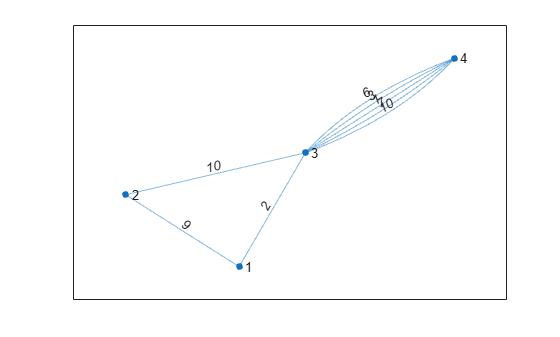```H_pick = simplify(G,'max'); plot(H_pick,'EdgeLabel',H_pick.Edges.Weight)```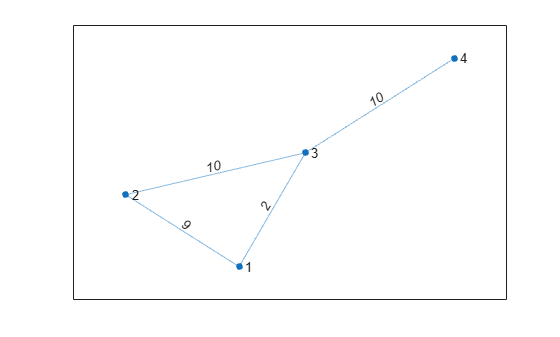```H_comb = simplify(G,'sum'); plot(H_comb,'EdgeLabel',H_comb.Edges.Weight)```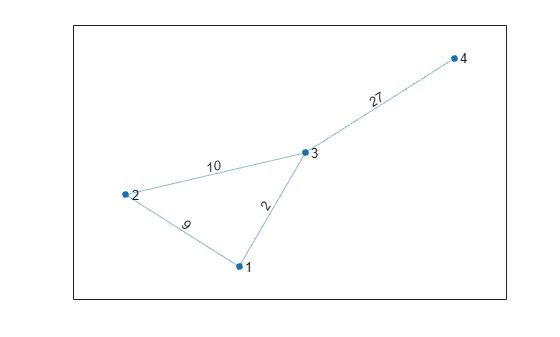```G = graph([1 1 1 1 1 1 1 2 2 2 2],[1 1 1 1 2 2 2 2 2 2 2 ]); plot(G) axis equal```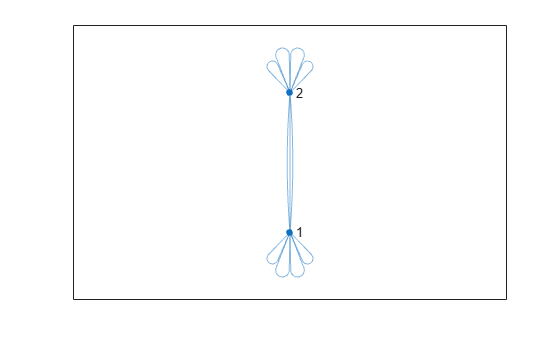```G = simplify(G,'keepselfloops'); plot(G) axis equal```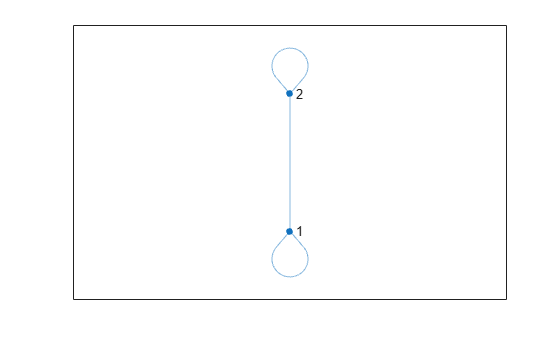```G = graph([1 1 1 2],[2 2 3 3]); G.Edges```
```ans=4×1 table EndNodes ________ 1 2 1 2 1 3 2 3 ```

`[G,ei,ec] = simplify(G)`
```G = graph with properties: Edges: [3x1 table] Nodes: [3x0 table] ```
```ei = 4×1 1 1 2 3 ```
```ec = 3×1 2 1 1 ```

`ei(i)` 是简化图中表示旧图中的边 `i` 的边。由于前两条边重复，因此 `ei(1) = ei(2) = 1`。而且 `ec(1) = 2`，因为新图中有两条边对应于旧图中的边 1。

```G = graph([1 1 1 1 1 1 2 2 2],[2 2 2 3 3 3 3 3 3],[],{'New York', 'Boston', 'Washington D.C.'}); G.Edges.Mode = categorical([1 2 3 1 2 3 1 2 3],[1 2 3],{'Air' 'Train' 'Bus'})'; G.Edges.Cost = [400 80 40 250 100 75 325 150 100]'; G.Edges.Time = [1 7 5 1.5 10 8 1.75 11 9]'; G.Edges```
```ans=9×4 table EndNodes Mode Cost Time ___________________________________ _____ ____ ____ {'New York'} {'Boston' } Air 400 1 {'New York'} {'Boston' } Train 80 7 {'New York'} {'Boston' } Bus 40 5 {'New York'} {'Washington D.C.'} Air 250 1.5 {'New York'} {'Washington D.C.'} Train 100 10 {'New York'} {'Washington D.C.'} Bus 75 8 {'Boston' } {'Washington D.C.'} Air 325 1.75 {'Boston' } {'Washington D.C.'} Train 150 11 {'Boston' } {'Washington D.C.'} Bus 100 9 ```

```plot(G,'EdgeLabel',cellstr(G.Edges.Mode),'LineWidth',G.Edges.Time./min(G.Edges.Time),'EdgeCData',G.Edges.Cost) colorbar```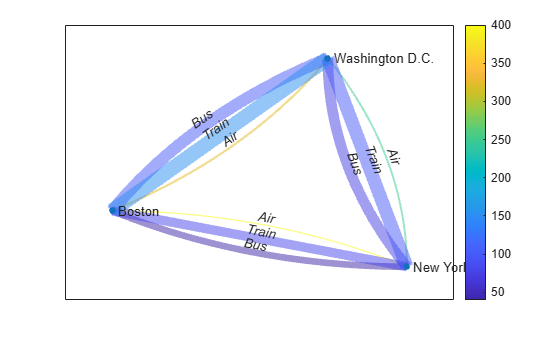```t = simplify(G,'min','PickVariable','Time'); plot(t,'EdgeLabel',cellstr(t.Edges.Mode))```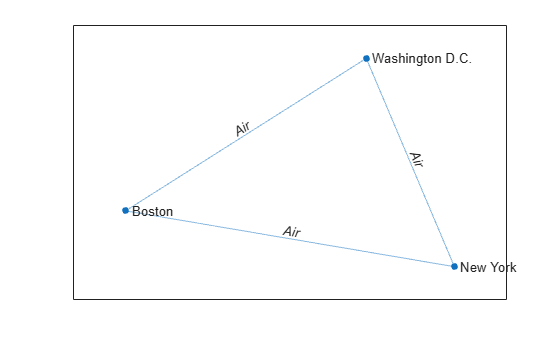```c = simplify(G,'sum','AggregationVariables','Cost'); plot(c,'EdgeLabel',c.Edges.Cost)```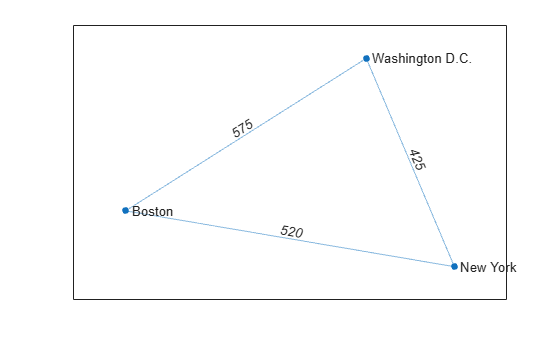## 输入参数

• 如果方法是 `'first'``'last'`，则 `simplify` 只保留边表 `G.Edges` 中出现的第一条边或最后一条边。

• 如果方法是 `'min'``'max'`，则 `simplify` 只保留具有最小权重或最大权重的边。`Weight` 变量必须存在于 `G.Edges` 中，除非您使用 `'PickVariable'` 名称-值对组基于不同的变量进行选择。

• `'omitselfloops'` - 删除图中的所有自环。这是默认设置。

• `'keepselfloops'` - 具有一个或多个自环的节点在简化图中只有一个自环。

### 名称-值参数

• 指定单个表变量名称的字符向量

• 字符向量元胞数组，其中每个元素表示一个表变量名称

• 指定一个或多个变量名称的字符串数组

• 表变量索引的向量

• 逻辑向量，每个元素分别对应一个表变量，其中 `true` 表示包含对应的变量，`false` 表示不包含对应的变量

• 函数句柄，以 `G.Edges` 表为输入并返回逻辑标量，如 `@isnumeric`